# Pime factor TreePage 1

#### WATCH ALL SLIDES

Slide 1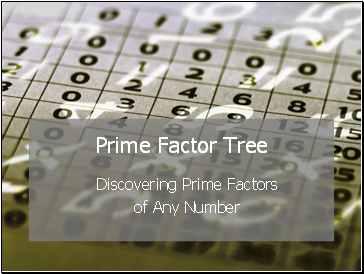Prime Factor Tree

Discovering Prime Factors

of Any Number

Slide 2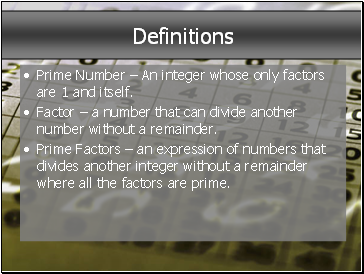## Definitions

Prime Number – An integer whose only factors are 1 and itself.

Factor – a number that can divide another number without a remainder.

Prime Factors – an expression of numbers that divides another integer without a remainder where all the factors are prime.

Slide 3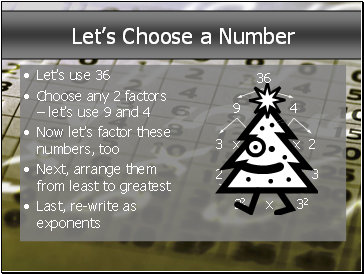## Let’s Choose a Number

Let’s use 36

Choose any 2 factors – let’s use 9 and 4

Now let’s factor these numbers, too

Next, arrange them from least to greatest

Last, re-write as exponents

36

Slide 4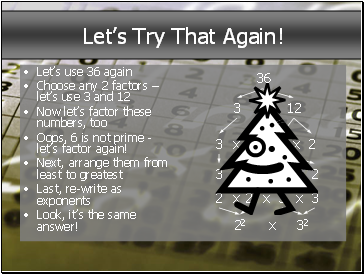## Let’s Try That Again!

Let’s use 36 again

Choose any 2 factors – let’s use 3 and 12

Now let’s factor these numbers, too

Oops, 6 is not prime - let’s factor again!

Next, arrange them from least to greatest

Last, re-write as exponents

36

Slide 5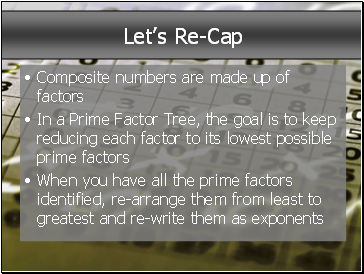## Let’s Re-Cap

Composite numbers are made up of factors

In a Prime Factor Tree, the goal is to keep reducing each factor to its lowest possible prime factors

When you have all the prime factors identified, re-arrange them from least to greatest and re-write them as exponents